# DTD Dyno Day at TDP

Saturday, February 13th, saw DTDIRL.com members attend a dyno day at TDP.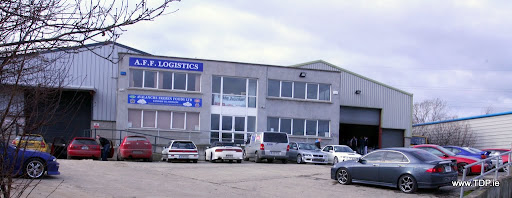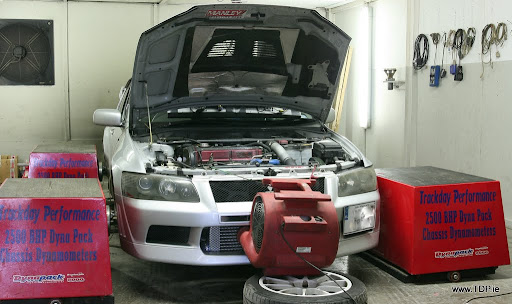1. Eoin – EVO VII GSR
This image shows the torque (lbft) on the left hand side of the page, and power (BHP) on the right hand side of the page, measured at the hubs. The torque figure is the ‘true torque’. It is calculated by: the torque produced at the flywheel multiplied by the gear ratio and the final drive ratio. The power figure is the power produced at the axles.This image shows the Flywheel Torque (lbft) on the left hand side of the page, and Flywheel Power (BHP) on the right hand side. As the title suggests, this is the torque and power that is produced at the flywheel. These figures are estimated and are correct to ± 5%.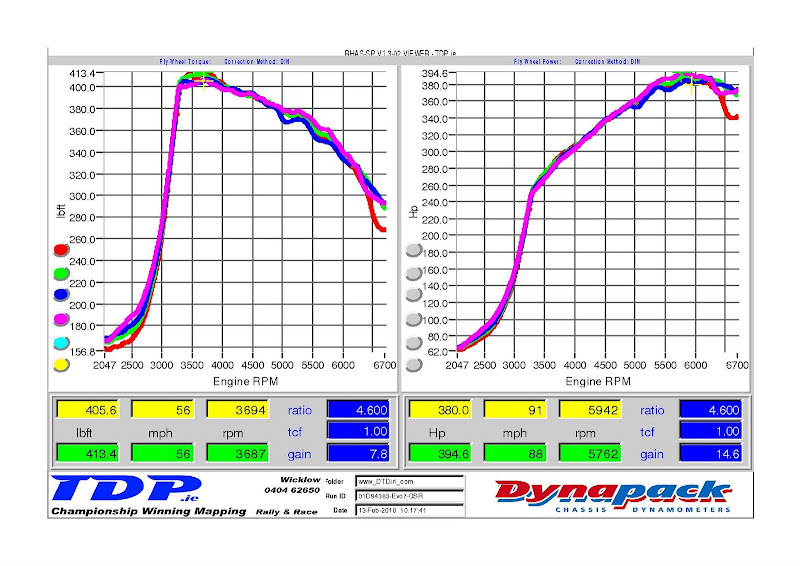This image shows the boost pressure.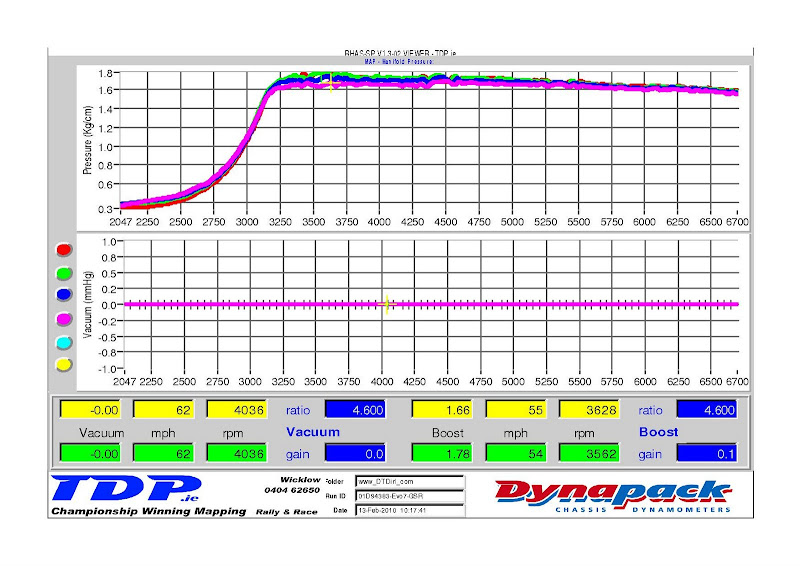2. Gary – RalliartEvo IV – Evo IV
This image shows the torque (lbft) on the left hand side of the page, and power (BHP) on the right hand side of the page, measured at the hubs. The torque figure is the ‘true torque’. It is calculated by: the torque produced at the flywheel multiplied by the gear ratio and the final drive ratio. The power figure is the power produced at the axles.This image shows the Flywheel Torque (lbft) on the left hand side of the page, and Flywheel Power (BHP) on the right hand side. As the title suggests, this is the torque and power that is produced at the flywheel. These figures are estimated and are correct to ± 5%.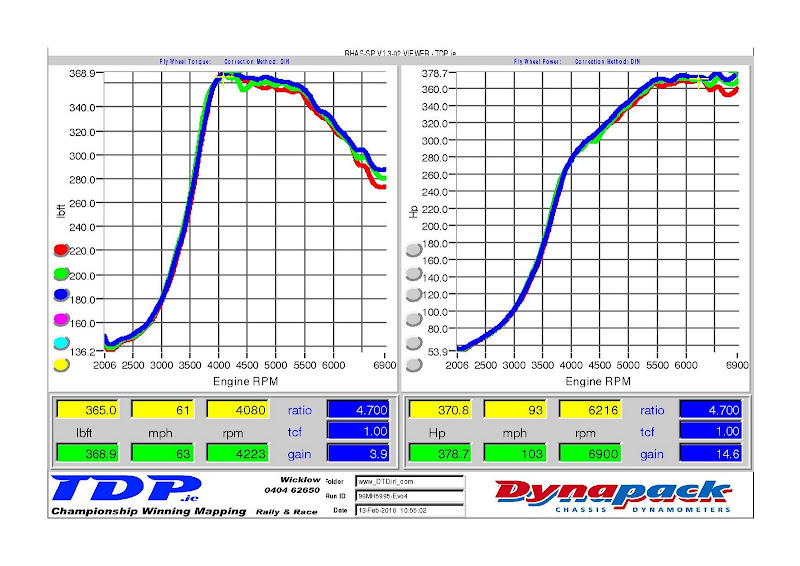This image shows the boost pressure.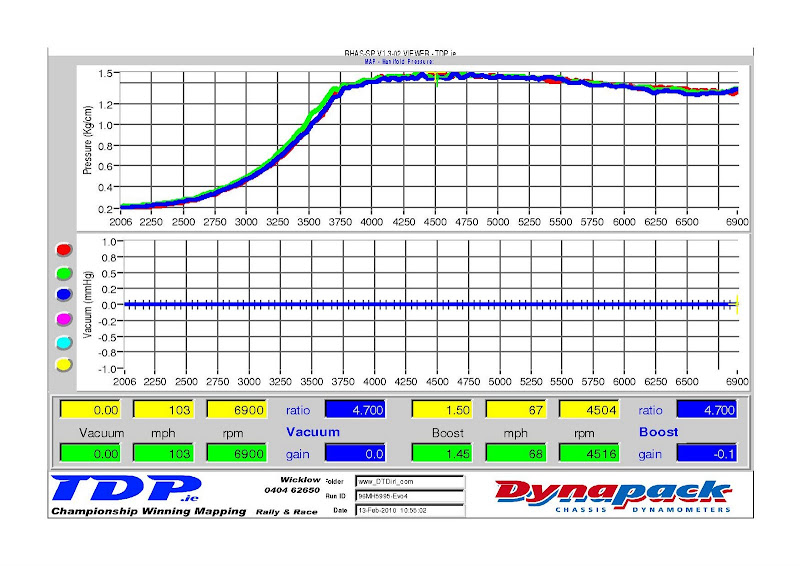3. Andy – Mr. Write Off – EVO V
This image shows the torque (lbft) on the left hand side of the page, and power (BHP) on the right hand side of the page, measured at the hubs. The torque figure is the ‘true torque’. It is calculated by: the torque produced at the flywheel multiplied by the gear ratio and the final drive ratio. The power figure is the power produced at the axles.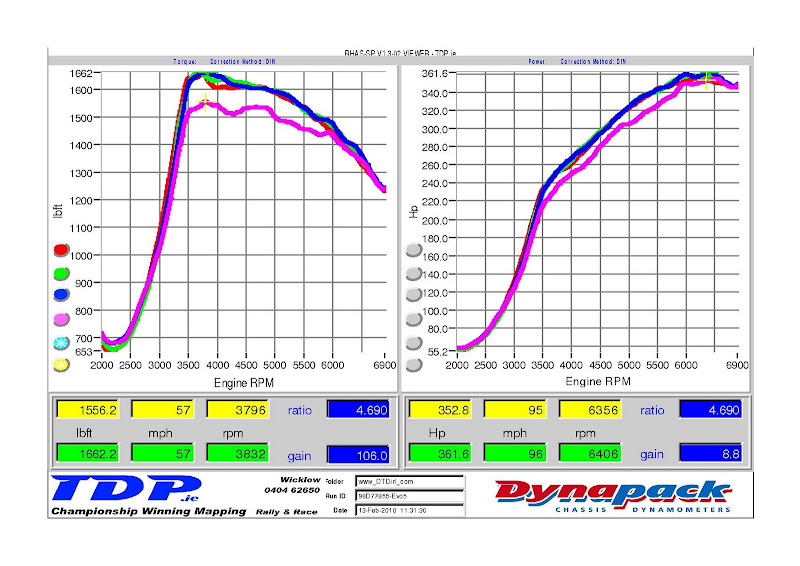This image shows the Flywheel Torque (lbft) on the left hand side of the page, and Flywheel Power (BHP) on the right hand side. As the title suggests, this is the torque and power that is produced at the flywheel. These figures are estimated and are correct to ± 5%.This image shows the boost pressure.4. Jacek – Krystian – EP3 Civic Type-R
This image shows the torque (lbft) on the left hand side of the page, and power (BHP) on the right hand side of the page, measured at the hubs. The torque figure is the ‘true torque’. It is calculated by: the torque produced at the flywheel multiplied by the gear ratio and the final drive ratio. The power figure is the power produced at the axles.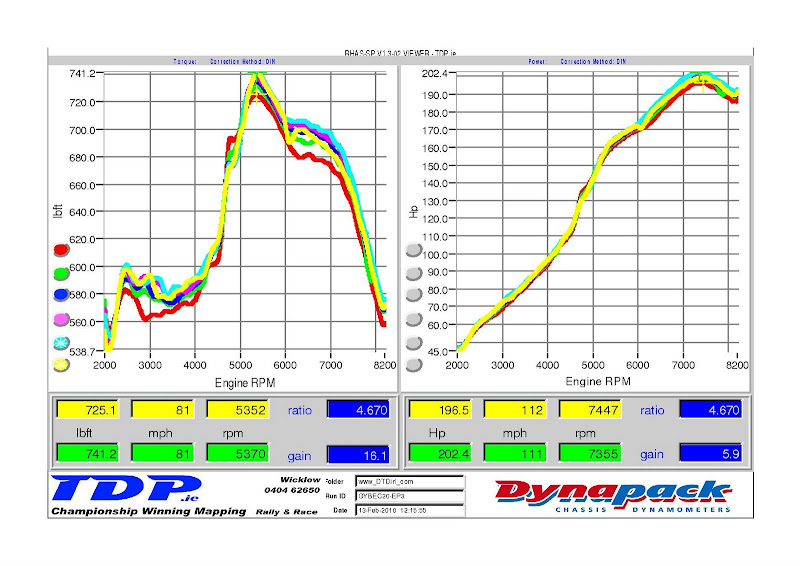This image shows the Flywheel Torque (lbft) on the left hand side of the page, and Flywheel Power (BHP) on the right hand side. As the title suggests, this is the torque and power that is produced at the flywheel. These figures are estimated and are correct to ± 5%.5. Ray – Ray Tsang – S15 Silvia
This image shows the torque (lbft) on the left hand side of the page, and power (BHP) on the right hand side of the page, measured at the hubs. The torque figure is the ‘true torque’. It is calculated by: the torque produced at the flywheel multiplied by the gear ratio and the final drive ratio. The power figure is the power produced at the axles.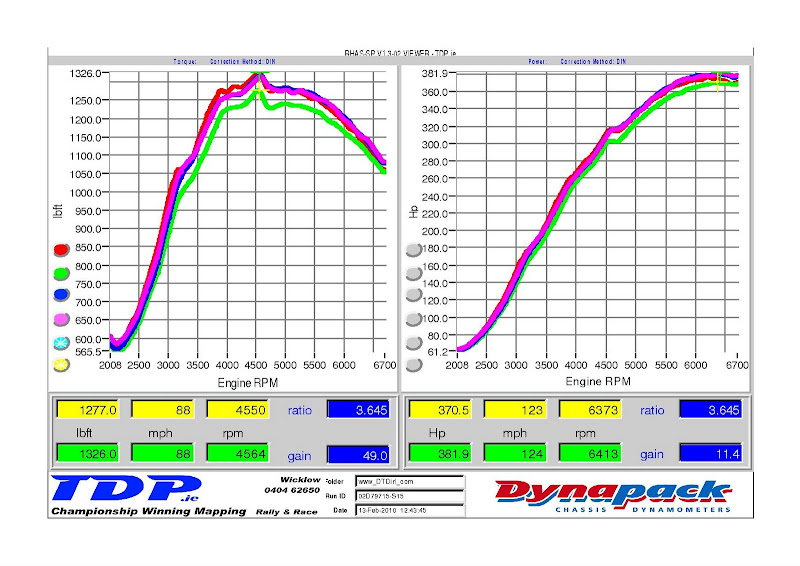This image shows the Flywheel Torque (lbft) on the left hand side of the page, and Flywheel Power (BHP) on the right hand side. As the title suggests, this is the torque and power that is produced at the flywheel. These figures are estimated and are correct to ± 5%.This image shows the boost pressure.6. Patrick – VTi Civic
This image shows the torque (lbft) on the left hand side of the page, and power (BHP) on the right hand side of the page, measured at the hubs. The torque figure is the ‘true torque’. It is calculated by: the torque produced at the flywheel multiplied by the gear ratio and the final drive ratio. The power figure is the power produced at the axles.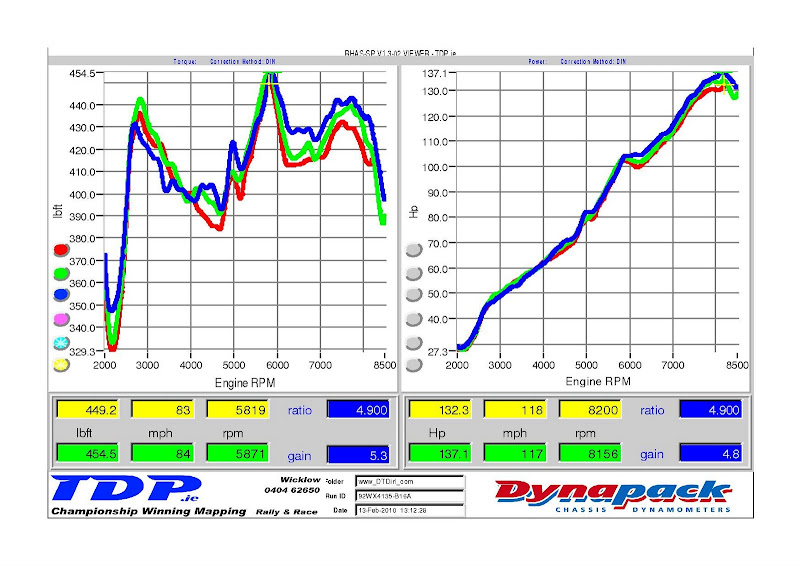This image shows the Flywheel Torque (lbft) on the left hand side of the page, and Flywheel Power (BHP) on the right hand side. As the title suggests, this is the torque and power that is produced at the flywheel. These figures are estimated and are correct to ± 5%.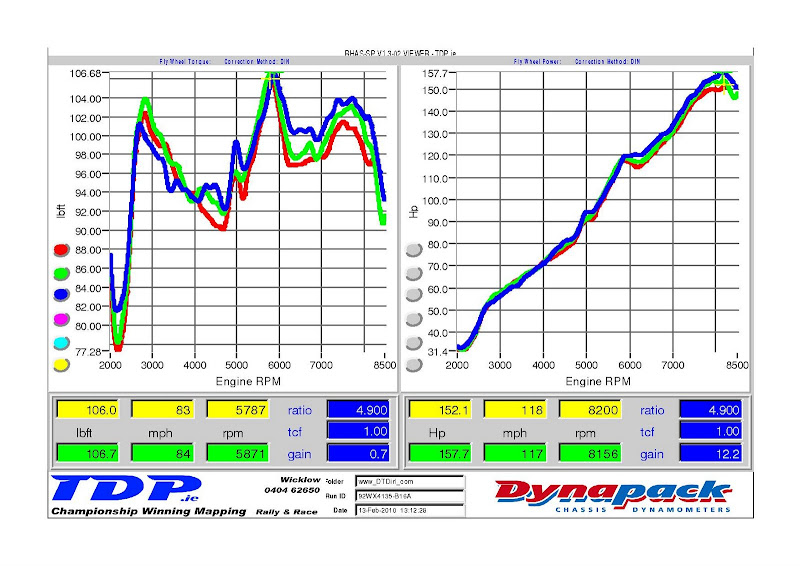7. Ian – Iand227 – Civic Jordan
This image shows the torque (lbft) on the left hand side of the page, and power (BHP) on the right hand side of the page, measured at the hubs. The torque figure is the ‘true torque’. It is calculated by: the torque produced at the flywheel multiplied by the gear ratio and the final drive ratio. The power figure is the power produced at the axles.This image shows the Flywheel Torque (lbft) on the left hand side of the page, and Flywheel Power (BHP) on the right hand side. As the title suggests, this is the torque and power that is produced at the flywheel. These figures are estimated and are correct to ± 5%.8. Diarmuid – Colt Mivec
This image shows the torque (lbft) on the left hand side of the page, and power (BHP) on the right hand side of the page, measured at the hubs. The torque figure is the ‘true torque’. It is calculated by: the torque produced at the flywheel multiplied by the gear ratio and the final drive ratio. The power figure is the power produced at the axles.This image shows the Flywheel Torque (lbft) on the left hand side of the page, and Flywheel Power (BHP) on the right hand side. As the title suggests, this is the torque and power that is produced at the flywheel. These figures are estimated and are correct to ± 5%.9. Gearóid – Turbo – 180SX
This car was running extremely lean, so we could only run it once on the dyno. At least the owner found out, before it was too late.
This image shows the torque (lbft) on the left hand side of the page, and power (BHP) on the right hand side of the page, measured at the hubs. The torque figure is the ‘true torque’. It is calculated by: the torque produced at the flywheel multiplied by the gear ratio and the final drive ratio. The power figure is the power produced at the axles.This image shows the Flywheel Torque (lbft) on the left hand side of the page, and Flywheel Power (BHP) on the right hand side. As the title suggests, this is the torque and power that is produced at the flywheel. These figures are estimated and are correct to ± 5%.10. Tommy – Leon 1.9 TDi
This image shows the torque (lbft) on the left hand side of the page, and power (BHP) on the right hand side of the page, measured at the hubs. The torque figure is the ‘true torque’. It is calculated by: the torque produced at the flywheel multiplied by the gear ratio and the final drive ratio. The power figure is the power produced at the axles.This image shows the Flywheel Torque (lbft) on the left hand side of the page, and Flywheel Power (BHP) on the right hand side. As the title suggests, this is the torque and power that is produced at the flywheel. These figures are estimated and are correct to ± 5%.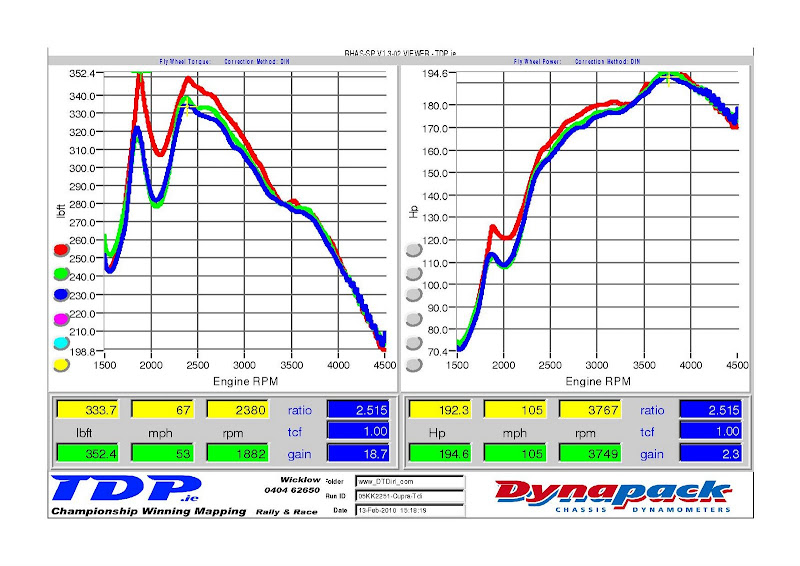This image shows the boost pressure.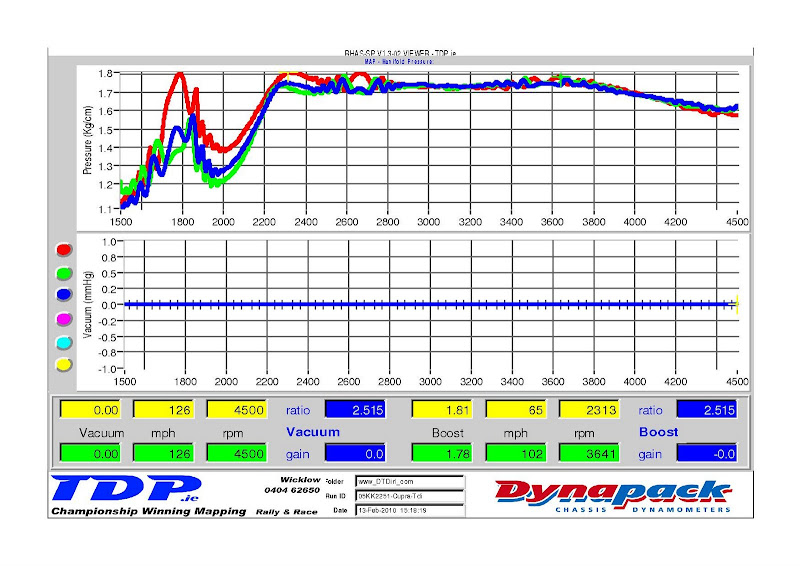11. Vivienne – xX Miss-Teg Xx – SiR Integra
This image shows the torque (lbft) on the left hand side of the page, and power (BHP) on the right hand side of the page, measured at the hubs. The torque figure is the ‘true torque’. It is calculated by: the torque produced at the flywheel multiplied by the gear ratio and the final drive ratio. The power figure is the power produced at the axles.This image shows the Flywheel Torque (lbft) on the left hand side of the page, and Flywheel Power (BHP) on the right hand side. As the title suggests, this is the torque and power that is produced at the flywheel. These figures are estimated and are correct to ± 5%.12. Mark – Coby – S14
This image shows the torque (lbft) on the left hand side of the page, and power (BHP) on the right hand side of the page, measured at the hubs. The torque figure is the ‘true torque’. It is calculated by: the torque produced at the flywheel multiplied by the gear ratio and the final drive ratio. The power figure is the power produced at the axles.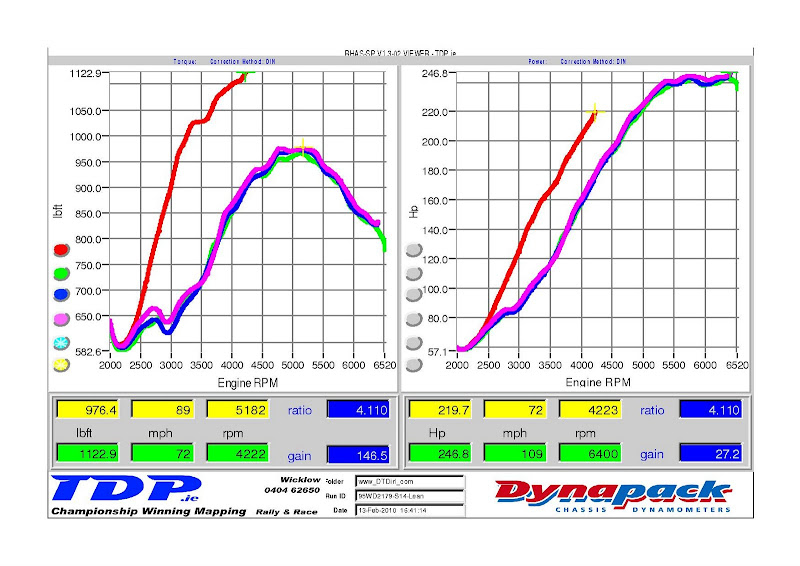This image shows the Flywheel Torque (lbft) on the left hand side of the page, and Flywheel Power (BHP) on the right hand side. As the title suggests, this is the torque and power that is produced at the flywheel. These figures are estimated and are correct to ± 5%.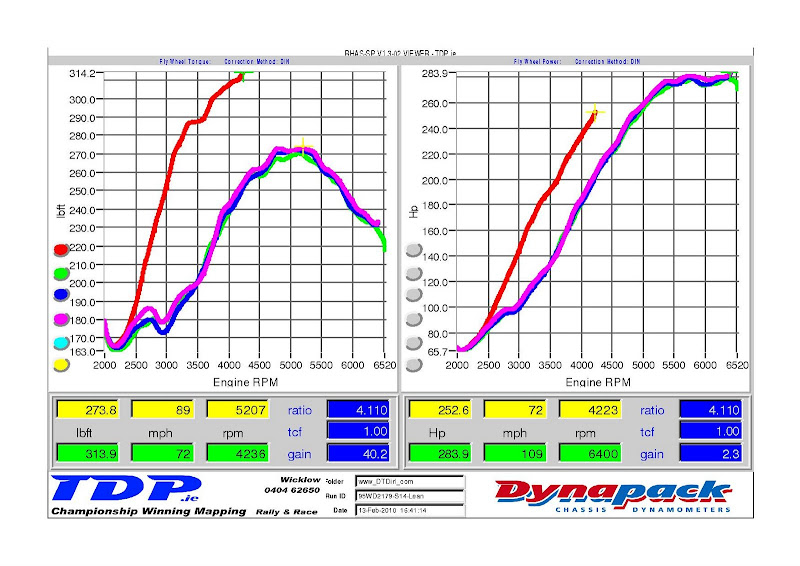This image shows the boost pressure.13. John – jstoneracing – Accord K24
This image shows the torque (lbft) on the left hand side of the page, and power (BHP) on the right hand side of the page, measured at the hubs. The torque figure is the ‘true torque’. It is calculated by: the torque produced at the flywheel multiplied by the gear ratio and the final drive ratio. The power figure is the power produced at the axles.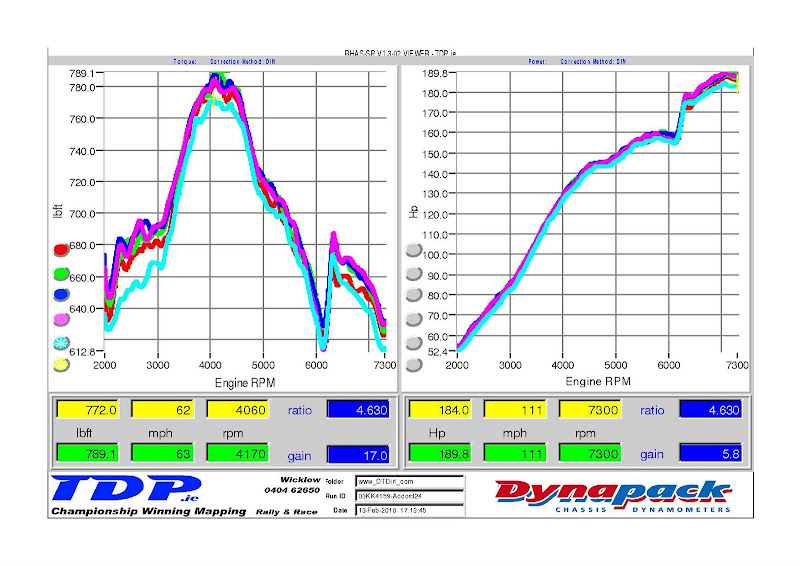This image shows the Flywheel Torque (lbft) on the left hand side of the page, and Flywheel Power (BHP) on the right hand side. As the title suggests, this is the torque and power that is produced at the flywheel. These figures are estimated and are correct to ± 5%.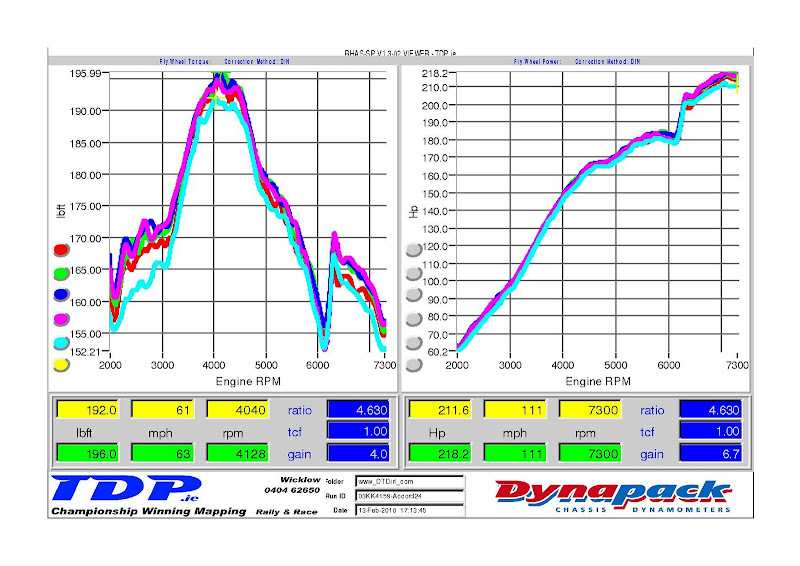14. Kevin – Supernova – Skyline R33 GTS-t
This image shows the torque (lbft) on the left hand side of the page, and power (BHP) on the right hand side of the page, measured at the hubs. The torque figure is the ‘true torque’. It is calculated by: the torque produced at the flywheel multiplied by the gear ratio and the final drive ratio. The power figure is the power produced at the axles.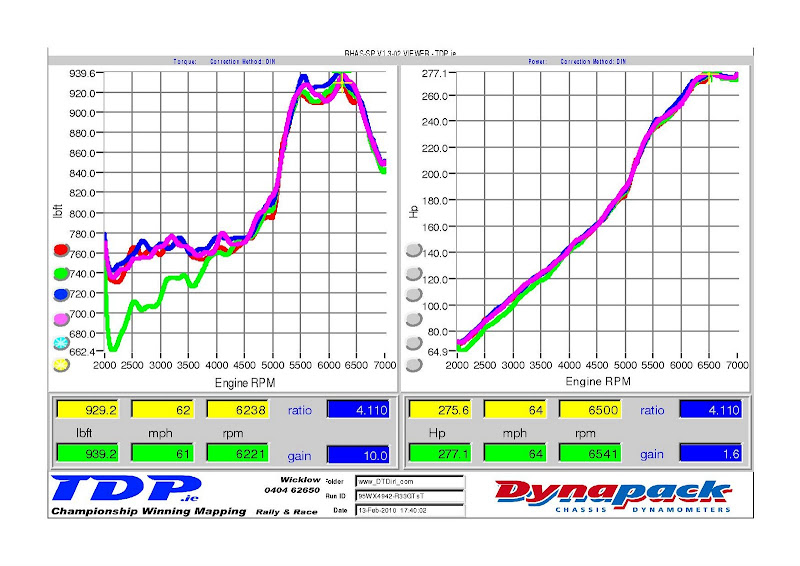This image shows the Flywheel Torque (lbft) on the left hand side of the page, and Flywheel Power (BHP) on the right hand side. As the title suggests, this is the torque and power that is produced at the flywheel. These figures are estimated and are correct to ± 5%.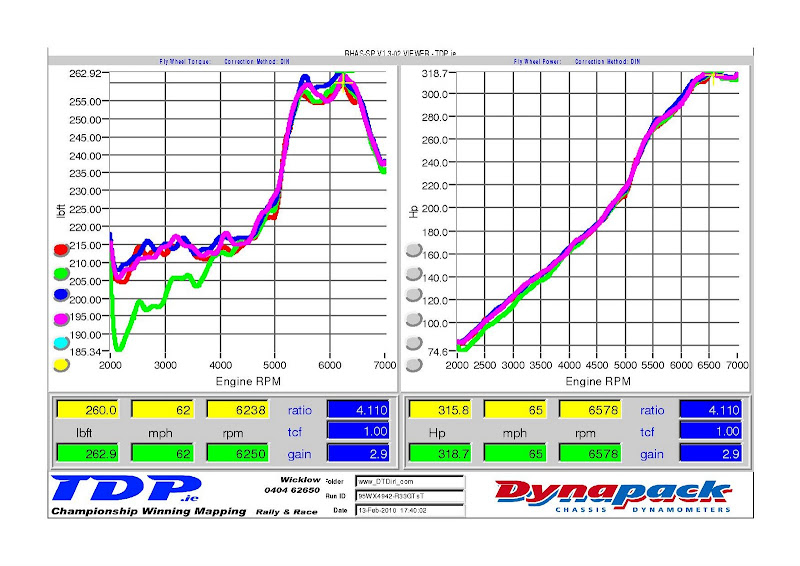This image shows the boost pressure.15. Alan – Civic – Apexi Neo set-up > Before and After Results
This image shows the torque (lbft) on the left hand side of the page, and power (BHP) on the right hand side of the page, measured at the hubs. The torque figure is the ‘true torque’. It is calculated by: the torque produced at the flywheel multiplied by the gear ratio and the final drive ratio. The power figure is the power produced at the axles.
The green line is before tuning, and the purple is after tuning.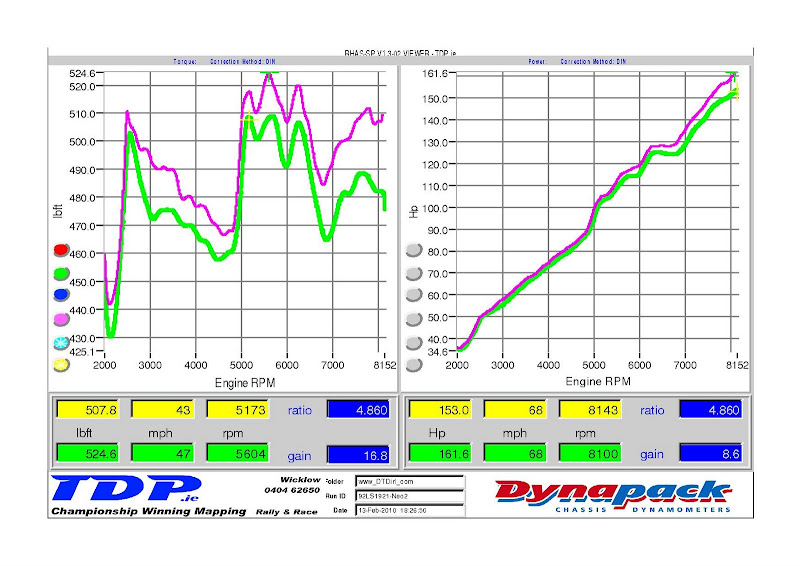This image shows the Flywheel Torque (lbft) on the left hand side of the page, and Flywheel Power (BHP) on the right hand side. As the title suggests, this is the torque and power that is produced at the flywheel. These figures are estimated and are correct to ± 5%.
The green line is before tuning, and the purple is after tuning.We’d like to give a big thank you to the DTD members in attendance. We hope you enjoyed your stay at TDP.